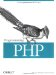3.6 Variable Functions

 As with variable variables, you can call a function based on the value of a variable. For example, consider this situation, where a variable is used to determine which of three functions to call: switch(\$which) { case 'first': first( ); break; case 'second': second( ); break; case 'third': third( ); break; } In this case, we could use a variable function call to call the appropriate function. To make a variable function call, include the parameters for a function in parentheses after the variable. To rewrite the previous example: \$which(); // if \$which is "first" the function first( ) is called, etc... If no function exists for the variable, a runtime error occurs when the code is evaluated. To prevent this, you can use the built-in function function_exists( ) to determine whether a function exists for the value of the variable before calling the function: \$yes_or_no = function_exists(function_name); For example: if(function_exists(\$which)) { \$which(); // if \$which is "first" the function first( ) is called, etc... } Language constructs such as echo( ) and isset( ) cannot be called through variable functions: \$f = 'echo'; \$f('hello, world'); // does not workProgramming PHP
ISBN: 1565926102
EAN: 2147483647
Year: 2007
Pages: 168

Similar book on Amazon